Coil Springs

Coil springs are the most common application of spring within motorsport. For reason why and some more information on coil springs make sure you check out our article “coil springs”.

There are two main ways to calculate spring rate. One is through calculations based upon looking at and measuring the spring. The other is by practical measurement. The practical measurement is the most accurate form when carried out with the correct equipment. Both ways are shown below.

The Calculation Route

The diagram below shows a coil spring along with the following important parameters that are required to calculate the spring rate.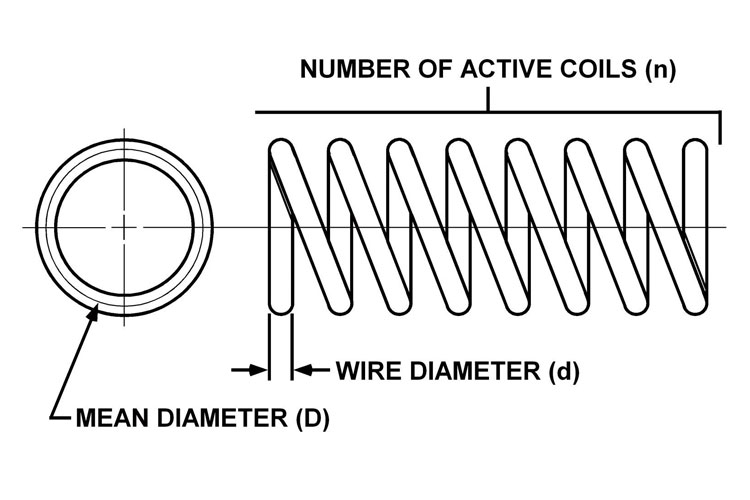The important parameters are:

• L = Free Length of The Unloaded Spring (m)
• G = Shear Modulus of Rigidity of Material
• d = Wire Diameter (m)
• D = Mean Diameter (m)
• N = Number of active coils (an active coil sweeps one full circle)

Where:

• Free length is the distance from the top face of the spring to the bottom face of the spring when no load is on it.
• Shear modulus of rigidity is based upon the type of material that the spring is made from. The value can be found in the table below. All you need to find out is what material your spring is made from. If you are unsure the most common material is highlighted in bold in the table.
 Material Shear Modulus of Rigidity (G) ANSI 1095 Spring Steel 79,300,000,000 Pa Cold Rolled Steel 75,000,000,000 Pa Stainless Steel 77,200,000,000 Pa
• Wire diameter is the thickness of the coil metal which is most accurately measured with vernier callipers
• Mean diameter is shown in the diagram and is the distance between centres of the coil spring. The easiest way to reach this number is with the below equation
• Mean Diameter = Total Spring Diameter – Wire Diameter
• The number of active coils still has uncertainty in the industry of how to apply an accurate number for a type of spring. The diagram below shows 4 common styles that a coil spring has at its ends.• Closed Ends
• Closed and Ground Ends
• Plain Ends Ground
• Plain Ends

The industry standard to now is that a spring with closed ends or closed and ground ends has one inactive coil at each end meaning that two coils have to be taken off the total amount of coils for the “number of active coils” parameter.

However, springs with plain ends are considered to have no inactive coils so every single coil counts towards the “number of active coils” parameter.

Finally, springs with plain ends ground are considered to have half an inactive coil at each end meaning that a total of 1 coil is removed for the “number of active coils” parameter.

It is very important to understand how your springs are finished as the number of active coils parameter can have a large influence on the calculated spring rate.

The Equation

With your measurements complete it is time to calculate the stiffness of your coil spring with the equation shown below.Therefore:So using the example figures of:

• G = 79.3GPa
• d = 10.3mm
• N = 6
• D = 68.5mmThe Practical Method

If you have access to some load testing equipment then the practical method is the most accurate option to calculate your spring rate. A machine such as a Tinnius-Olsen shown below is the ideal piece of equipment for this test.  If you have access to one or something similar then insert your spring on the machine and compress it by 10mm. Record the force required to compress it at this point. Then compress the spring in stages of 10mm, recording the force required at each point. If the spring starts to become over-stressed towards the end of the test then do not keep compressing as it could damage the spring.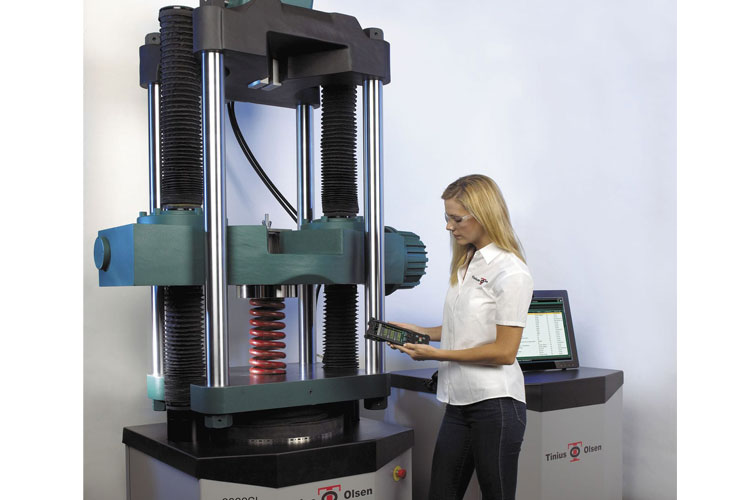With all of your results in a similar format to the below example, convert all your millimetre readings to meters. Then divide the force required by the distance moved in each case. If all of the answers to this look similar then you have a constant rate spring. You can now add up all of the answers and divide it by the number of results to get the average reading which is your spring rate.

If the answers get progressively smaller or larger by a noticeable amount then you have a progressive rate spring. If this is the case for you it would be best to plot a graph of your results n excel tracing spring rate against mm of compression. This will be very important information to know when applying pre-load to your spring. Also, if you know how much your car lowers when it is sat on its wheels then you can calculate the static spring rate of your springs at ride height for future reference.

Leaf SpringsCalculating the spring rate for a leaf spring is much more complex than for a coil spring. This is due to the number of variables that can apply to leaf springs such as; leaf thickness, width and taper, end constraint variations or the load being applied off centre etc. Therefore the most accurate way to measure leaf spring stiffness is practically. However, for a close answer you can also use the calculation route where some approximations have to be made.

The Calculation Route

There are two main types of leaf spring within automotive applications. They are “single leaf parabolic” and “laminated leaf spring”. The latter is more common in modern applications. Images below display the different types.

Single Leaf Parabolic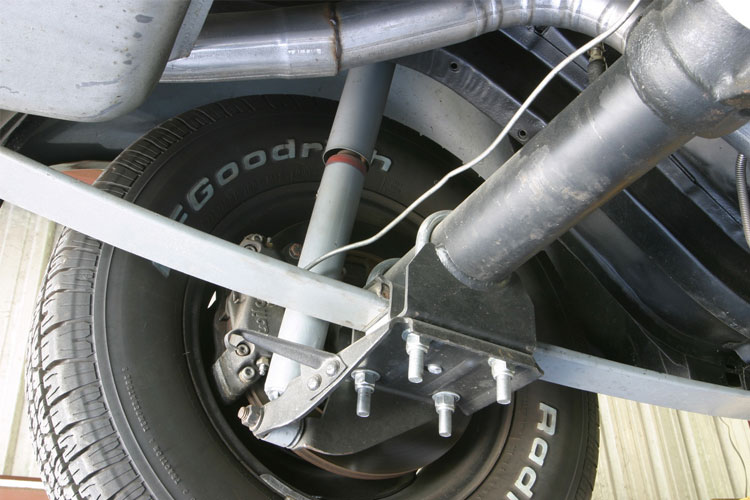Laminated Leaf SpringTwo equations apply for leaf springs. One is the bending stress formula to ensure that maximum load will not over stress the material. The other is the spring stiffness. This is the figure that is important for further calculations. The equations for a single leaf parabolic spring are:And:Where:

• L = Half the overall length of the longest leaf spring (m)
• F = Force applied at each mounting point to the chassis (usually half the load applied at the axle point) (m)
• b = Leaf spring width at the centre point (m)
• t = Vertical depth of the leaf spring at the centre point where it mounts to the axle (m)
• E = Youngs modulus for the material (Pa) (see table below)
• X = Spring displacement vertically (m)

The equations for a laminated leaf spring vary slightly and are:And: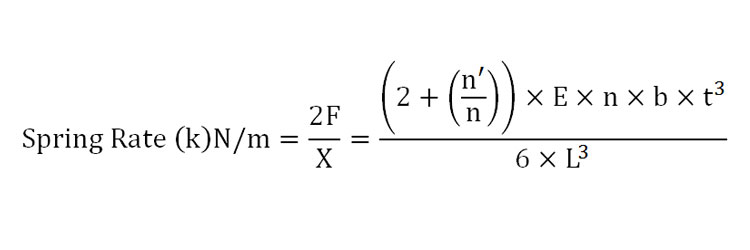Where:

• L = Half the overall length of the longest leaf spring (m)
• F = Force applied at each mounting point to the chassis (usually half the load applied at the axle point) (m)
• b = Leaf spring width at the centre point (m)
• n = number of leaves stacked
• n’ = number of leaves directly at the spring ends
• t = Vertical depth of the leaf spring at the centre point where it mounts to the axle (m)
• E = Youngs modulus for the material (Pa) (see table below)
• X = Spring displacement vertically (m)

Youngs Modulus Table for Common Materials

 Material Youngs Modulus (E) ANSI 1095 Spring Steel 207,000,000,000 Pa Cold Rolled Steel 186,000,000,000 Pa Mild Steel 210,000,000,000 Pa

The Practical Route

The more accurate route to measure the stiffness of your leaf springs is to test them practically if you have the correct load application equipment. To test the load you need to disconnect the axle form the spring and move it away from directly below the spring. Next, a load needs applying using a device that will measure the amount of load being applied in Newtons of force. The leaf spring needs to be deflected in steps of 10mm increments with the force required to move the spring being recorded. For each step the force can be divided by the displacement to give the spring rate using the below equation. If the numbers have a large variation and increase each time after the below equation has been used then the spring has a progressive rate and a graph should be plotted in excel to show what rate is present at each point of displacement as this will be more accurate than using an equation.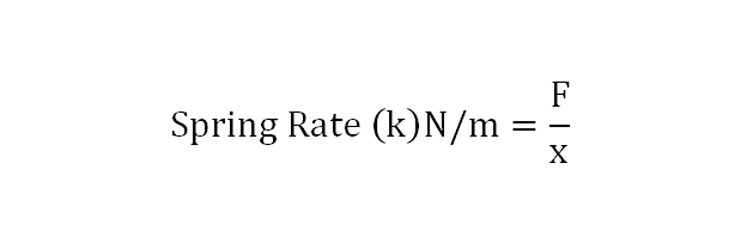Where:

• F = Force applied (N)
• x = Amount of displacement (m)

How to Convert Metric to Imperial

If you would rather have your spring rates in terms of pounds and inches then you can use the below conversion equation to change the result form newtons per meter into pounds per inch.Likewise if you wish to convert pounds per inch into newtons per meter then input the pounds per inch value where it says example below and I will produce the newtons per meter answer.How to Add Spring Rates for Multiple Springs

There are two configurations that multiple springs come in. One is springs in series and one is springs in parallel. A car can be considered to have springs in parallel because if you look at the front axis of a car each wheel as its own spring acting on the front of the vehicle making a total of two springs working side by side. This makes them parallel.

Springs in Series

A few examples are shown below of when a spring can be considered in series.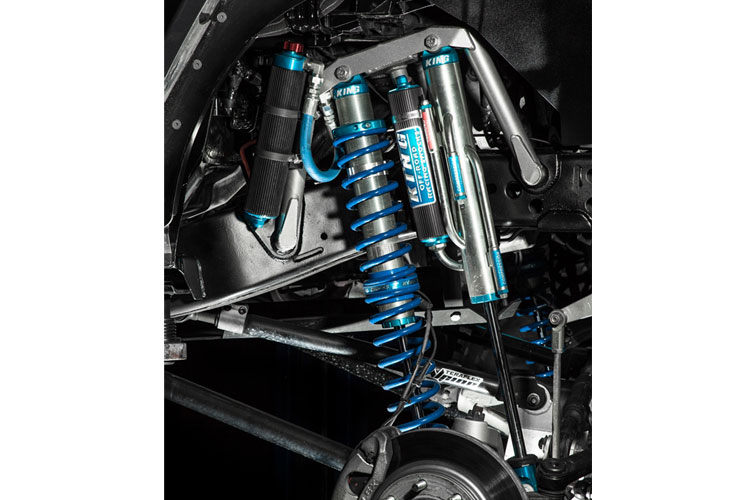When two springs or more are put on top of each other, the combined spring rate always becomes less than that of the softest spring. This is because you have effectively added even more coils to the softer spring (N) which reduces overall spring rate. The spring rate for each individual spring must first be known before the below equation can be used to calculate the total spring rate of the springs in series. If two springs are being used in series then the below equation can be used:Where:

• K total = Combined spring rate
• K1 = bottom spring rate
• K2 = Top spring rate

If more than two springs are in series then the next spring up can keep being added to the equation for all the springs; for example in the case of 4 springs stacked on top of each other the equation would be like the below: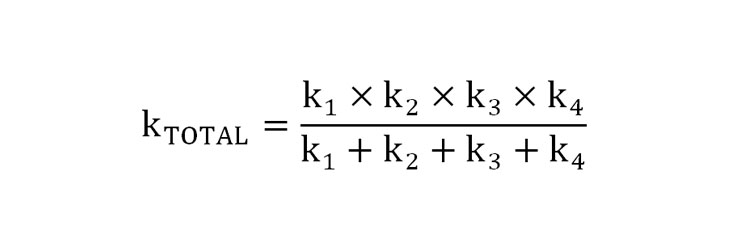Springs in Parallel

Springs in parallel can be achieved in a few ways as well. The images below show a few examples of when springs can be considered in parallel.Springs are said to be in parallel when they always share a load. The composite rate of parallel springs is much easier to calculate than springs in series as the spring rates are simply added together. The equation below can be used to calculate the overall effective spring rate of the parallel springs:And so on.

Before the release of part 2 next week please also read “How to Adjust and Tune Anti-Roll Bars” for information on how to calculate anti-roll bar spring rates.

##### Similar Posts

1.Rob

Re. coil springs, photos or drawings of the various spring ends (closed or closed & ground etc.) would help clarify which is which.

1.Suspension Secrets

Hi Rob,

Thanks very much for your feedback. we will add these to the post shortly.

Many Thanks

Suspension Secrets

2.Derek

Can you confirm that the spring rate equation for a laminated leaf spring is correct? I am getting answers that are roughly 1/6 the value of other calculators.

1.Suspension Secrets

Hi Derek, thanks for your comment. Yes we have checked it multiple times now and it also compares directly to multiple publications. It is important to ensure that all units are being inputted correctly as SI units and the brackets are being used correctly as these things can hugely affect the result.

Thanks
Suspension Secrets